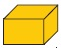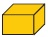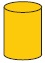# User Forum

Subject :IMO    Class : Class 4+= 70 g and the total weight of 10, 10and 10is 3200 g, then what is the weight of?

A 250 g
B 190 g
C 210 g
D 320 g

a

Class : Class 5
A

Class : Class 4

## Ans 3:

Class : Class 4
10 big rectangle,10 small rectangle & 10 cylinder = 3200. Then 1 big rectangle + 1 small rectangle + 1 cylinder = 3200 divided by 10 = 320. Big rectangle + small rectangle = 70g. Then 320 -70 =250g A